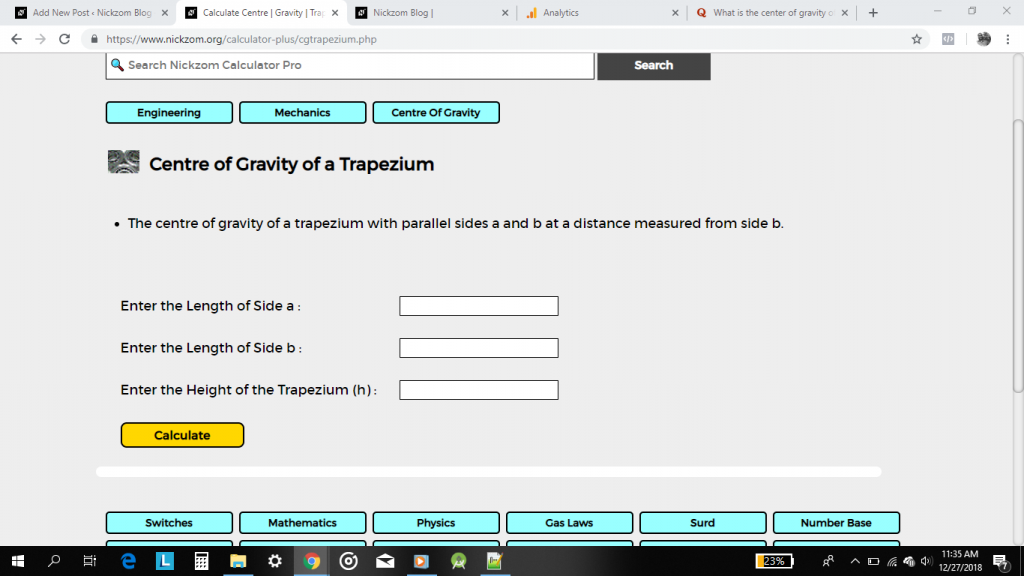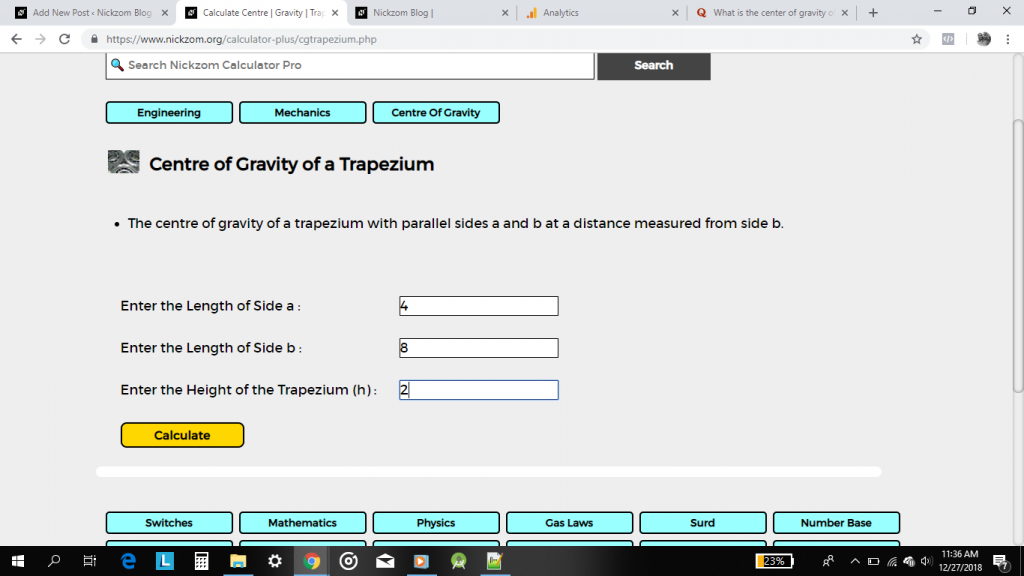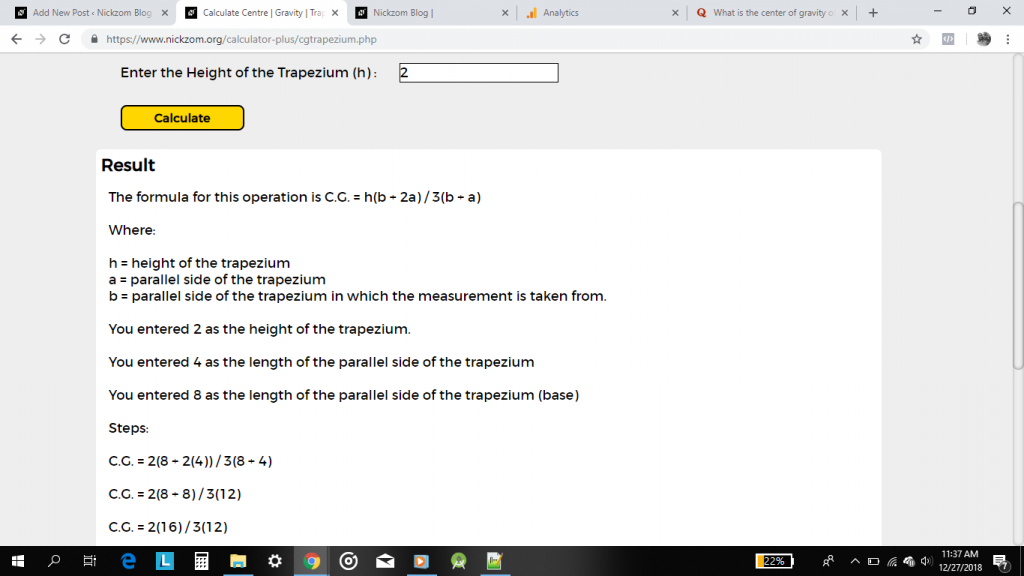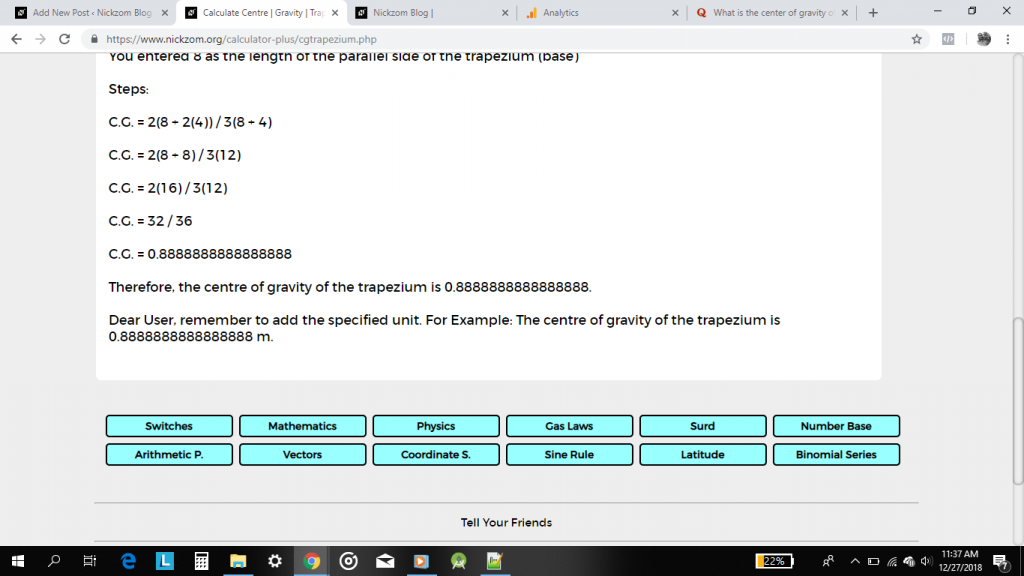# Nickzom Calculator Calculates the Center of Gravity of a Trapezium in Statics Mechanics

According to Quora,

The center of gravity of a trapezoid can be estimated by dividing the trapezoid in two triangles.

Nickzom Calculator calculates the centre of gravity of a trapezium with parallel sides a and b at a distance measured from side b.

The formula for calculating this center of gravity is:

C.G. = h (b + 2a) / 3 (b + a)

Where, a and b are the length of the parallel sides of the trapezium (b being the base length and a being the top length) whereas h is the height of the trapezium.

Let’s take for Example: Find the center of gravity or centroid of a trapezium where a is 4, b is 8 and h is 2.

This implies:

a = 4
b = 8
h = 2

C.G. = 2 (8 + 2(4)) / 3 (8 + 4)
C.G. = 2 (8 + 8) / 3 (12)
C.G. = 2 (16) / 36
C.G. = 32 / 36
C.G. = 0.89

Therefore, the center of gravity or centroid of the trapezium is 0.89.

To get the answer and workings to center of gravity or centroid of a trapezium. First, you need to obtain the Nickzom Calculator – The Calculator Encyclopedia app.

You can get this app via any of these means:

Once, you have obtained the calculator encyclopedia app, proceed to the Calculator Map, then click on Mechanics under the Engineering sectionNow, click on Centre of Gravity under StaticsNow, click on Centre of Gravity of a TrapeziumThe screenshot below displays the page to enter your values to get answers for centre of gravity of a trapezium according to the respective parameters.Now, enter the values accordingly like in the example aboveNow, click on CalculateYou can see that Nickzom Calculator solves to the precise answer while still displaying the steps.